test for Quick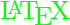At first, we sample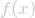in the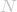(is odd) equidistant points around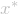:whereis some step.
Then we interpolate points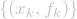by polynomial

(1)Its coefficientsare found as a solution of system of linear equations:

(2)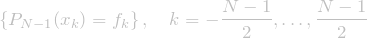Here are references to existing equations: (1), (2).
Here is reference to non-existing equation (??).

Mathematical graphs: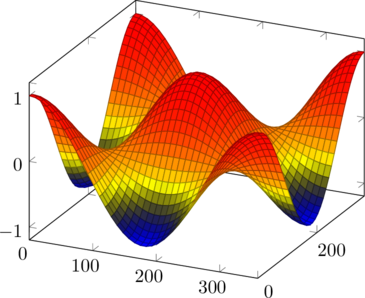几种$\LaTeX$插件比较

Comparison of several  &  plugins.

1.Easy LaTeX 1.42
http://wordpress.org/extend/plugins/easy-latex/
+公式格式可设置为三种格式之一：$$…!$$ $…!$
$$…!$$：内嵌公式格式
$$!…!$$：独立行居中格式
$$…!!$$：显示公式原文
+解析标题
+可设置cache，cache的位置在安装目录easy-latex下的cache中
+可以设置前景色和背景色，但背景色无法设置为透明
+认识TeX和LaTeX的logo

2.Latex for WordPress 2.01
http://wordpress.org/extend/plugins/latex/
+公式格式：$$…!$$
$$…!$$：内嵌公式格式
$$!…!$$：独立行居中格式
$$…!!$$：显示公式原文
+解析标题
*不可设置前景色和背景色，背景色自动设置为透明
*强制要求在wp-content下设置名为cache的缓冲目录，需要设置可写权限
+认识TeX和LaTeX的logo

3.WP QuickLaTeX
http://wordpress.org/extend/plugins/wp-quicklatex/

+公式有错误的时候会给出具体的错误信息
+解析标题
-不认识TeX和LaTeX的logo

4.WP LaTeX 1.7
http://wordpress.org/extend/plugins/wp-latex/

-不解析标题
+可设置前景色和背景色，背景色可以设置为透明
+认识TeX和LaTeX的logo

5.Youngwhan’s Simple Latex

-不解析标题
-不可设置颜色，背景色自动为透明
+可设置Location of mathtex.cgi，也就是说可以在你自己的独立主机上运行
+认识TeX和LaTeX的logo

-不解析标题
+认识TeX和LaTeX的logo

Easy LaTeX和Latex for WordPress相比，前者唯一的缺点是不能设置背景色为透明，后者不能设置前景色而背景色自动设置为透明。前者的缓存目录在插件自身的目录中，后者需要建立一个独立的cache目录，时间久了可能会忘了这是什么目录。

http://www.forkosh.dreamhost.com/mathtex.cgi

http://www.openmaths.org/cgi-bin/mathtex.cgi

http://www.quantnet.com/cgi-bin/mathtex.cgi

http://zhiqiang.org/blog/plugin/mimetex